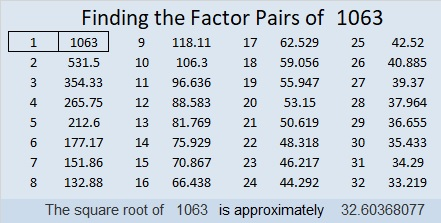# 1063 and Level 1

Lucky you found this puzzle today! You can solve it by writing the factors 1 to 12 in both the first column and the top row so that the given clues are the products of the corresponding factors.Print the puzzles or type the solution in this excel file: 12 factors 1063-1072

Now I’ll share a little information about the number 1063:

• 1063 is a prime number. 1061 and 1063 are twin primes.
• Prime factorization: 1063 is prime.
• The exponent of prime number 1063 is 1. Adding 1 to that exponent we get (1 + 1) = 2. Therefore 1063 has exactly 2 factors.
• Factors of 1063: 1, 1063
• Factor pairs: 1063 = 1 × 1063
• 1063 has no square factors that allow its square root to be simplified. √1063 ≈ 32.60368

How do we know that 1063 is a prime number? If 1063 were not a prime number, then it would be divisible by at least one prime number less than or equal to √1063 ≈ 32.6. Since 1063 cannot be divided evenly by 2, 3, 5, 7, 11, 13, 17, 19, 23, 29 or 31, we know that 1063 is a prime number.1063 is the sum of seven consecutive prime numbers:
137 + 139 + 149 + 151 + 157 + 163 + 167 = 1063

1063 is a high flying palindrome in one other base:
It’s 747 in BASE 12 because 7(12²) + 4(12) + 7(1) = 1063

This site uses Akismet to reduce spam. Learn how your comment data is processed.